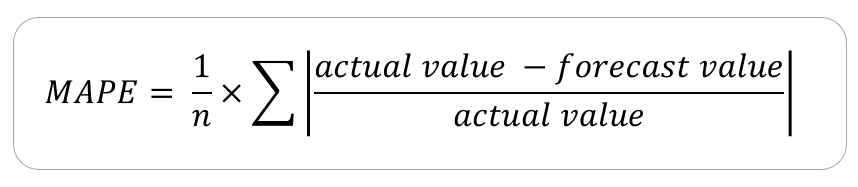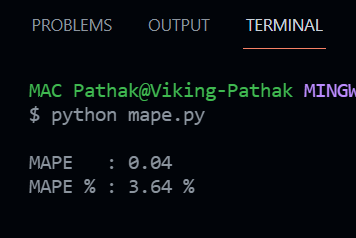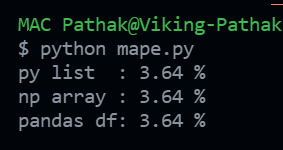GFG App
Open AppBrowser
Continue

In this article, we will see how to compute one of the methods to determine forecast accuracy called the Mean. Absolute Percentage Error (or simply MAPE) also known as Mean Absolute Percentage Deviation (MAPD) in python. The MAPE term determines how better accuracy does our forecast gives. The ‘M’ in MAPE stands for mean which takes in the average value over a series, ‘A’ stands for absolute that uses absolute values to keep the positive and negative errors from canceling one another out, ‘P’ is the percentage that makes this accuracy metric a relative metric, and the ‘E’ stands for error since this metric helps to determine the amount of error our forecast has.

Consider the following example, where we have the sales information of a store. The day column represents the day number which we are referring to, the actual sales column represents the actual sales value for the respective day whereas the forecast sales column represents the forecasted values for the sales figures (probably with an ML model). The APE column stands for Absolute percentage error (APE) which represents the percentage error between the actual and the forecasted value for the corresponding day. The formula for the percentage error is (actual value – forecast value) / actual value. The APE is the positive (absolute) value of this percentage error

Now, the MAPE value can be found by taking the mean of the APE values. The formula can be represented as –MAPE formula

## Python

 `# Define the dataset as python lists ` `actual   ``=` `[``136``, ``120``, ``138``, ``155``, ``149``] ` `forecast ``=` `[``134``, ``124``, ``132``, ``141``, ``149``] ` ` `  `# Consider a list APE to store the ` `# APE value for each of the records in dataset ` `APE ``=` `[] ` ` `  `# Iterate over the list values ` `for` `day ``in` `range``(``5``): ` ` `  `    ``# Calculate percentage error ` `    ``per_err ``=` `(actual[day] ``-` `forecast[day]) ``/` `actual[day] ` ` `  `    ``# Take absolute value of ` `    ``# the percentage error (APE) ` `    ``per_err ``=` `abs``(per_err) ` ` `  `    ``# Append it to the APE list ` `    ``APE.append(per_err) ` ` `  `# Calculate the MAPE ` `MAPE ``=` `sum``(APE)``/``len``(APE) ` ` `  `# Print the MAPE value and percentage ` `print``(f``''' ` `MAPE   : { round(MAPE, 2) } ` `MAPE % : { round(MAPE*100, 2) } % ` `'''``)`

Output:MAPE Output – 1

MAPE output is a non-negative floating-point. The best value for MAPE is 0.0 whereas a higher value determines that the predictions are not accurate enough. However, how much large a MAPE value should be to term it as an inefficient prediction depends upon the use case. In the above output, we can see that the forecast values are good enough because the MAPE suggests that there is a 3% error in the forecasted values for the sales made on each day.

If you are working on time series data in python, you might be probably working with pandas or NumPy. In such case, you can use the following code to get the MAPE output.

## Python

 `import` `pandas as pd ` `import` `numpy as np ` ` `  `# Define the function to return the MAPE values ` `def` `calculate_mape(actual, predicted) ``-``> ``float``: ` ` `  `    ``# Convert actual and predicted ` `    ``# to numpy array data type if not already ` `    ``if` `not` `all``([``isinstance``(actual, np.ndarray), ` `                ``isinstance``(predicted, np.ndarray)]): ` `        ``actual, predicted ``=` `np.array(actual),  ` `        ``np.array(predicted) ` ` `  `    ``# Calculate the MAPE value and return ` `    ``return` `round``(np.mean(np.``abs``(( ` `      ``actual ``-` `predicted) ``/` `actual)) ``*` `100``, ``2``) ` ` `  `if` `__name__ ``=``=` `'__main__'``: ` ` `  `    ``# CALCULATE MAPE FROM PYTHON LIST ` `    ``actual    ``=` `[``136``, ``120``, ``138``, ``155``, ``149``] ` `    ``predicted ``=` `[``134``, ``124``, ``132``, ``141``, ``149``] ` ` `  `    ``# Get MAPE for python list as parameters ` `    ``print``(``"py list  :"``, ` `          ``calculate_mape(actual, ` `                         ``predicted), ``"%"``) ` ` `  `    ``# CALCULATE MAPE FROM NUMPY ARRAY ` `    ``actual    ``=` `np.array([``136``, ``120``, ``138``, ``155``, ``149``]) ` `    ``predicted ``=` `np.array([``134``, ``124``, ``132``, ``141``, ``149``]) ` ` `  `    ``# Get MAPE for python list as parameters ` `    ``print``(``"np array :"``,  ` `          ``calculate_mape(actual, ` `                         ``predicted), ``"%"``) ` ` `  `    ``# CALCULATE MAPE FROM PANDAS DATAFRAME ` `     `  `    ``# Define the pandas dataframe ` `    ``sales_df ``=` `pd.DataFrame({ ` `        ``"actual"`    `: [``136``, ``120``, ``138``, ``155``, ``149``], ` `        ``"predicted"` `: [``134``, ``124``, ``132``, ``141``, ``149``] ` `    ``}) ` ` `  `    ``# Get MAPE for pandas series as parameters ` `    ``print``(``"pandas df:"``,  ` `          ``calculate_mape(sales_df.actual,  ` `                         ``sales_df.predicted), ``"%"``) `

Output:MAPE Output – 2

In the above program, we have depicted a single function `calculate_mape()` which does the MAPE calculation for a given python list, NumPy array, or pandas series. The output is the same as the same data is passed to all the 3 data type formats as parameters to the function.

My Personal Notes arrow_drop_up